# MATH 124: FINAL EXAMINATIONDUE AT 5PM ON SUNDAY, JANUARY 13TH, 2001

William Stein

Date: Math 124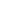HARVARD UNIVERSITYFall 2001

Instructions. Thoroughly justify all answers. While taking the exam, please do not discuss these problems with anyone else, and consult only those references that are explicitly mentioned on the Math 124 web page. (If you inadvertently stumble upon a solution while reading or studying for another class, e.g., Math 122, please make that clear in your solution.)

If you wish to use the result of a homework problem in the solution of one of the problems below, include your solution of that homework problem (a photocopy is acceptable). You may use any result that was proved in the lecture notes or the course textbooks, but please give a precise reference.

There are 10 problems, each worth 10 points, and problem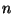was inspired'' by homework assignment. Problems 3, 5(ii), 6, 7, and 10 would be difficult to do without a computer; for these problems, you do not have to use PARI, though I recommend that you do.

Turning in the exam. The exam is due on Sunday, January 13th at 5pm. Some of you don't have a key to the math department, so you might not be able to go to my office (SC 515) and give me your exam. If this is the case, call me at (617)495-1790 or (617)308-0144, and I'll come downstairs and meet you at the elevator, and if that doesn't work just slide it under my door on Monday morning (but no later!!).

1.
The Fibonnaci numbers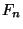are defined as follows: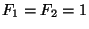and for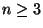,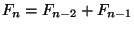. Prove that for every integer, the greatest common divisor ofand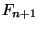is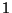.

2.
Letbe an odd positive integer, and let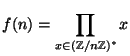, where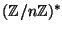is the group of integers modulothat are coprime to.
(i)
(5 points) Prove that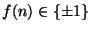.
(i)
(5 points) Find a formula for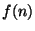in terms of the number of prime factors of. [Hint: You might find the result of Problem 4 on this exam useful.]

3.
Consider the RSA cryptosystem with public key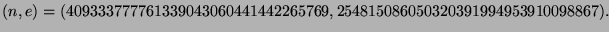(i)
(3 points) A secret message has been encoded as the number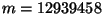. Encrypt the number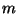using the above RSA cryptosystem.
(i)
(4 points) Find the decoding key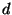; i.e., break'' this RSA cryptosystem.
(i)
(3 points) Decrypt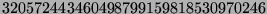. [Hint: The answer won't look like total nonsense.]

4.
Let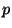be an odd prime. Prove that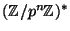is cyclic for all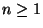. (I.e., prove that there is an element ofof order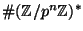.)

5.
(i)
(5 points) Evaluate the infinite continued fraction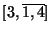.
(i)
(5 points) Determine the infinite continued fraction of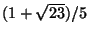.

6.
Write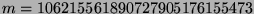as a sum of two positive integer squares.

7.
Determine the structure of the group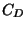of equivalence classes of primitive positive definite binary quadratic forms of discriminant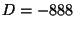. Your answer should consist ofexpressed as a product of cyclic groups. [Hint: Use the functions in forms.gp from Lecture 24.]

8.
This problem describes a special case of a theorem that was originally proven by Eichler and Shimura. The modularity theorem says that a similar statement is true for every elliptic curve over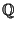.
(i)
(3 points) Let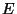be the elliptic curve given by the equation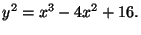Let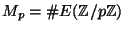be the number of points onover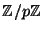(don't forget the point at infinity). Calculate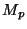for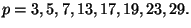(i)
(3 points) Let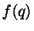be the (formal) power series given by the infinite product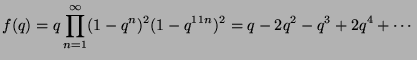Let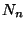be the coefficient of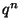in,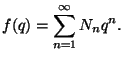Calculatefor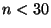.
(i)
(4 points) For each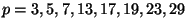, compute the sum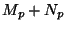of the quantities calculated in (i) and (ii), then formulate a conjecture as to what this value should be for any prime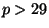.

9.
Letbe the elliptic curve defined by the equation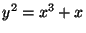. For each odd prime, let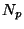be the number of points in the group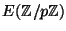of points onwith coordinates in.
(i)
(5 points) For each odd prime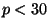, find.
(i)
(5 points) Make a general conjecture for the value ofwhen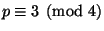, and prove your conjecture.

10.
Demonstrate how to use the elliptic curve factorization method to completely factor the integer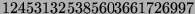. (You may use the isprime function of PARI to verify primality of numbers.)

11.
(Extra credit: automatic A in course, plus fame and glory) Letbe the elliptic curve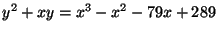. Prove that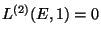, where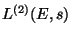is the second derivative of the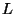-series associated to.